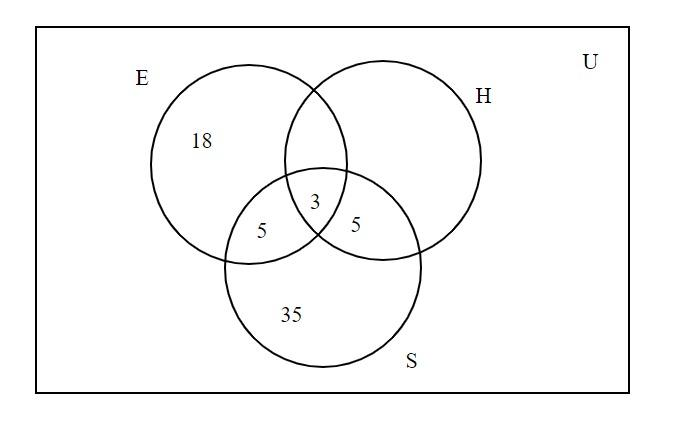Questions & AnswersQuestionAnswers

# In a survey of 100 students, the number of students studying the various languages were found to be: English only 18, English but not Hindi 23, English and Sanskrit 8, English 26, Sanskrit 48, Sanskrit and Hindi 8, no language 24. Find how many students were studying Hindi?Answer Verified
Hint: In this question, first draw the Venn diagram it will give us a clear picture of what we have to find out. Then you can easily know how many students are studying all the languages. So, use this concept to reach the solution of the question.

Complete step-by-step answer:
Let U denotes the set of surveyed students i.e., $n\left( U \right) = 100$
Again, let E, H and S denote the set of students who are studying English, Hindi and Sanskrit respectively.

Given,
Number of students studying English $n\left( E \right) = 26$
Number of students studying Sanskrit $n\left( S \right) = 48$
Number of students studying English and Sanskrit $n\left( {E \cap S} \right) = 8$
Number of students studying Sanskrit and Hindi $n\left( {S \cap H} \right) = 8$
Number of students studying no language $n(NL) = 24$Clearly from the Venn diagram,
Number of students studying language is $n\left( {E \cup H \cup S} \right) = n\left( U \right) - n\left( {NL} \right) = 100 - 24 = 76$
Number of students studying all the languages i.e., English, Sanskrit and Hindi $n\left( {E \cap H \cap S} \right) = 3$
Number of students studying only Hindi and Sanskrit = 5
So,
The number of students studying Hindi only $= \left\{ {n\left( U \right) - \left( {18 + 5 + 3 + 5 + 35} \right)} \right\} - n\left( {NL} \right)$
$= \left\{ {100 - \left( {66} \right)} \right\} - 24 \\ = 34 - 24 \\ = 10 \\$
Therefore
Number of students studying Hindi = number of students studying Hindi only + number of students
Studying only Hindi and Sanskrit + $n\left( {E \cap H \cap S} \right)$
= 10+5+3
= 18
Thus, the number of students studying Hindi is 18.

Note: In this problem the number of total students is always equal to the sum of number of students studying languages and number of students studying no language. Always draw the Venn diagram in the universal set.
Bookmark added to your notes.
View Notes
Nomenclature of Elements with Atomic Number above 100Farewell Speech For StudentsFarewell Speech for College StudentsFactors of a NumberTable of 100 - Multiplication Table of 100Factors of 100Conjugate of a Complex NumberCensus and Sample SurveyImportance of Sports Essay for Students in EnglishStatus of Women in India Essay for Students in English